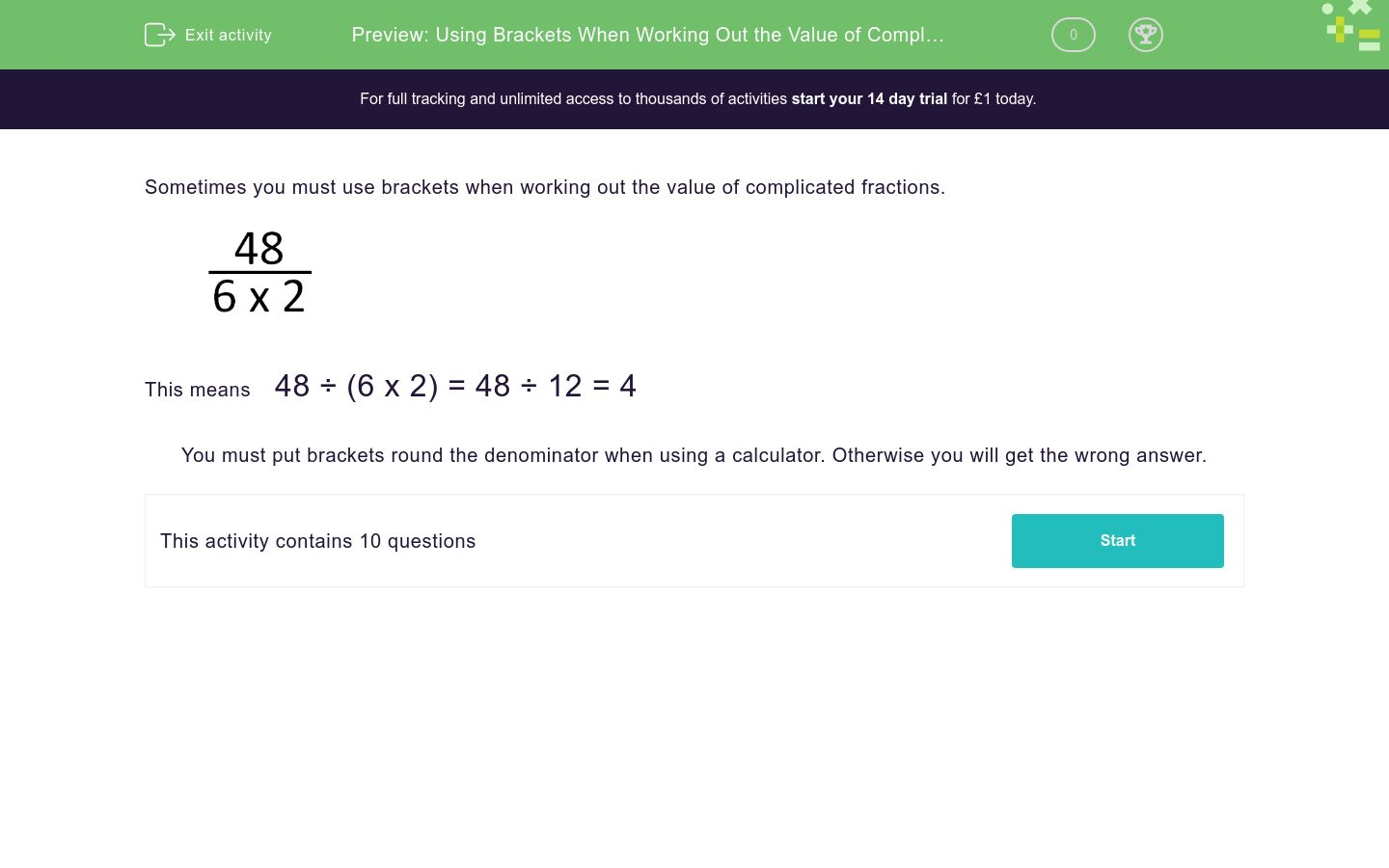# Using Brackets When Working Out the Value of Complex Fractions

In this worksheet, students use brackets when working out the value of more complicated fractions.Key stage:  KS 3

Curriculum topic:   Number

Curriculum subtopic:   Use Four Operations for All Numbers

Difficulty level:### QUESTION 1 of 10

Sometimes you must use brackets when working out the value of complicated fractions.This means    48 ÷ (6 x 2) = 48 ÷ 12 = 4

You must put brackets round the denominator when using a calculator. Otherwise you will get the wrong answer.

Find the value:

 40 2 × 4
= _____________

Find the value:

 45 2 + 3
= _____________

Find the value:

 80 4 × 2
= _____________

Find the value:

 90 6 + 4
= _____________

Find the value:

 50 6 + 4
= _____________

Find the value:

 99 7 + 4
= _____________

Find the value:

 98 4 + 3
= _____________

Find the value:

 64 2 + 2
= _____________

Find the value:

 60 10 + 2
= _____________

Find the value:

 51 8 + 9
= _____________
• Question 1

Find the value:

 40 2 × 4
= _____________
5
• Question 2

Find the value:

 45 2 + 3
= _____________
9
• Question 3

Find the value:

 80 4 × 2
= _____________
10
• Question 4

Find the value:

 90 6 + 4
= _____________
9
• Question 5

Find the value:

 50 6 + 4
= _____________
5
• Question 6

Find the value:

 99 7 + 4
= _____________

9
• Question 7

Find the value:

 98 4 + 3
= _____________
14
• Question 8

Find the value:

 64 2 + 2
= _____________
16
• Question 9

Find the value:

 60 10 + 2
= _____________
5
• Question 10

Find the value:

 51 8 + 9
= _____________
3
---- OR ----

Sign up for a £1 trial so you can track and measure your child's progress on this activity.

### What is EdPlace?

We're your National Curriculum aligned online education content provider helping each child succeed in English, maths and science from year 1 to GCSE. With an EdPlace account you’ll be able to track and measure progress, helping each child achieve their best. We build confidence and attainment by personalising each child’s learning at a level that suits them.

Get started